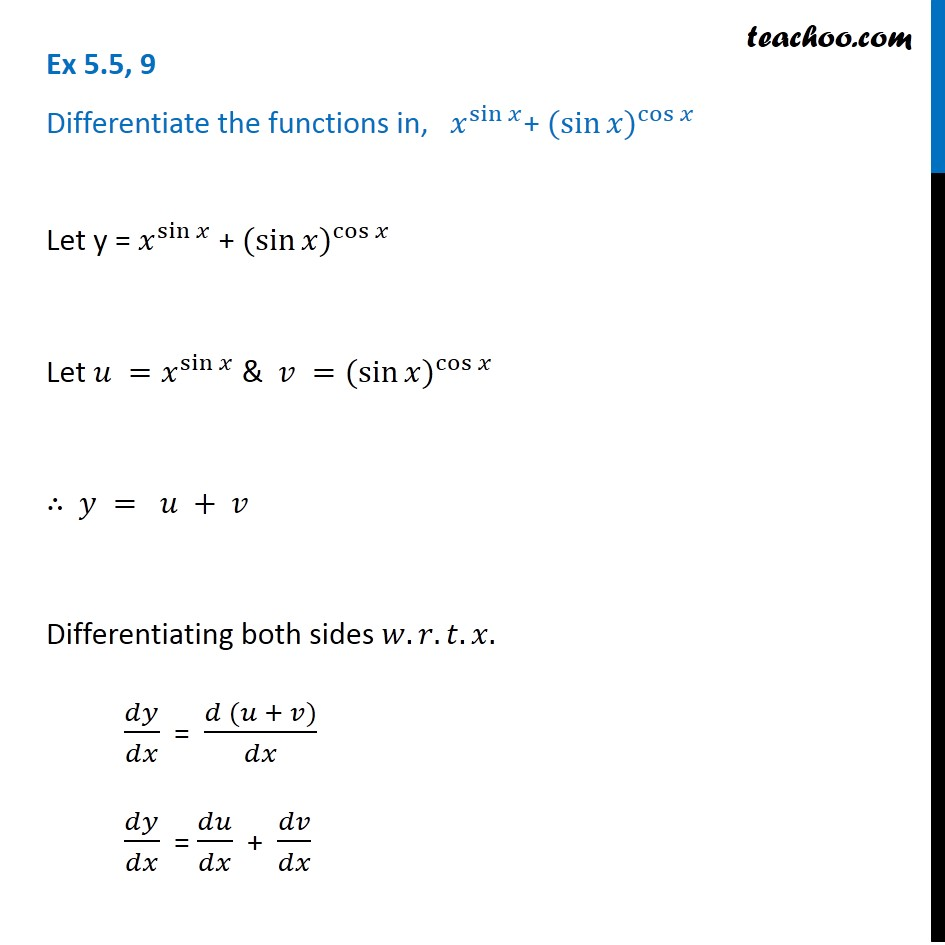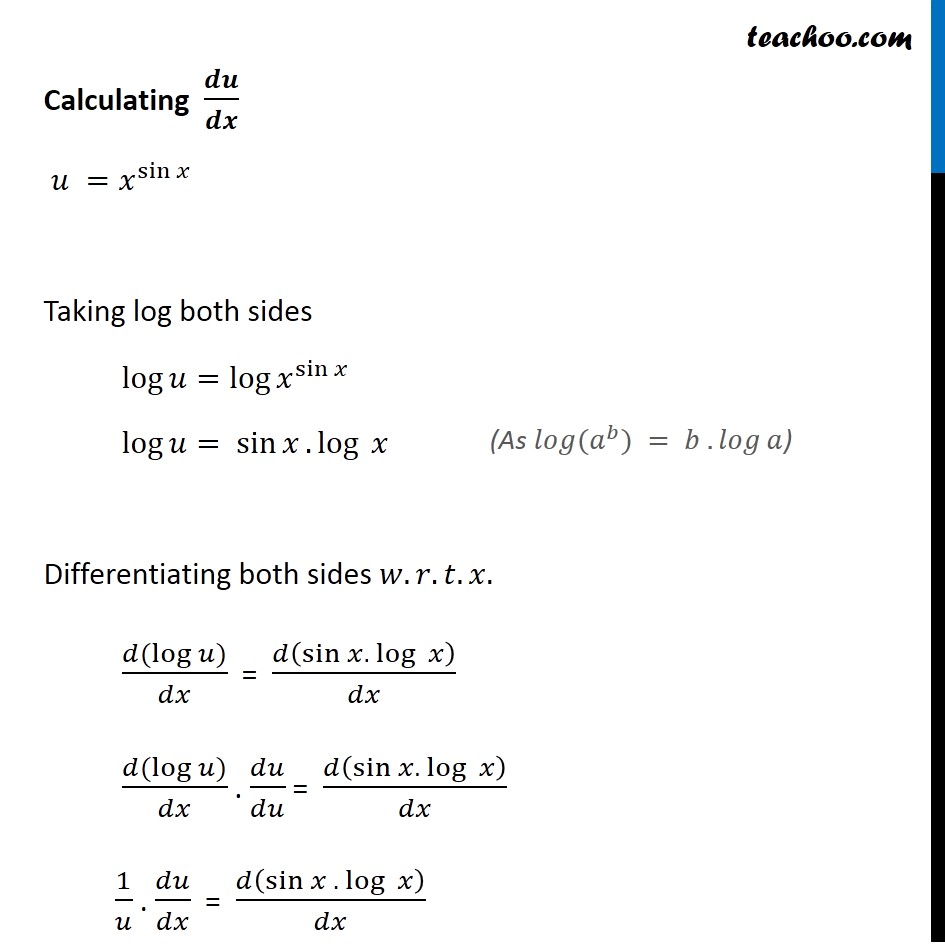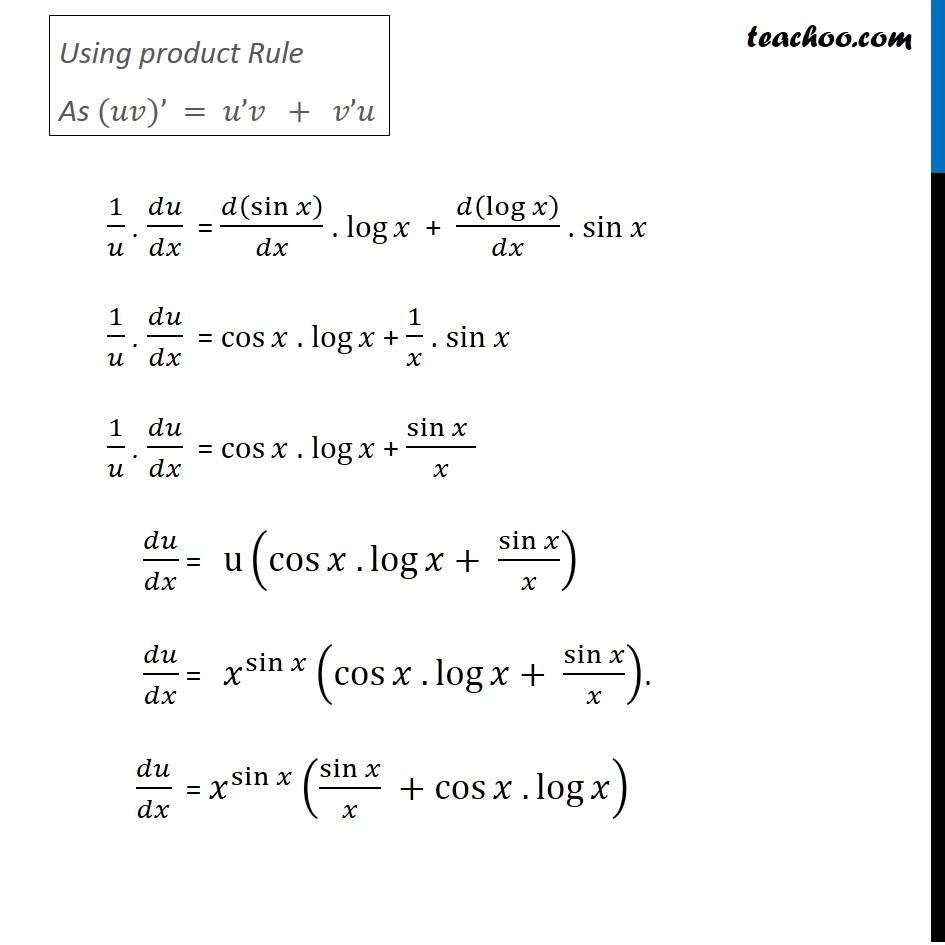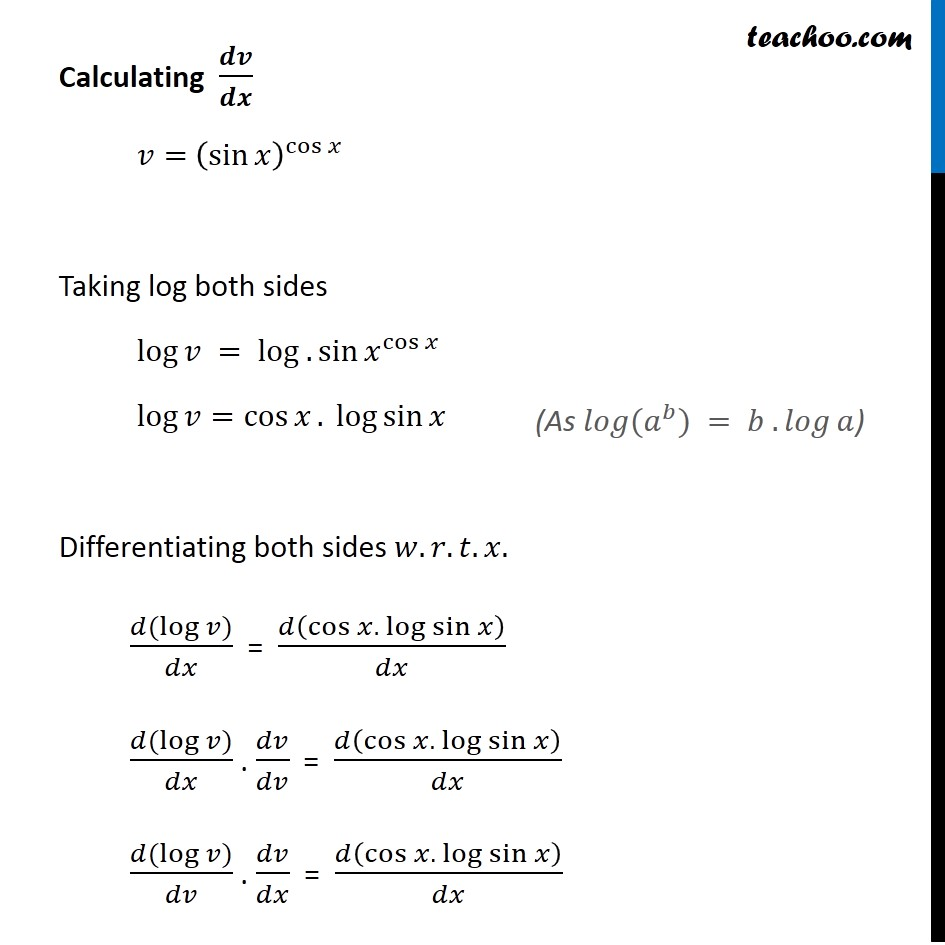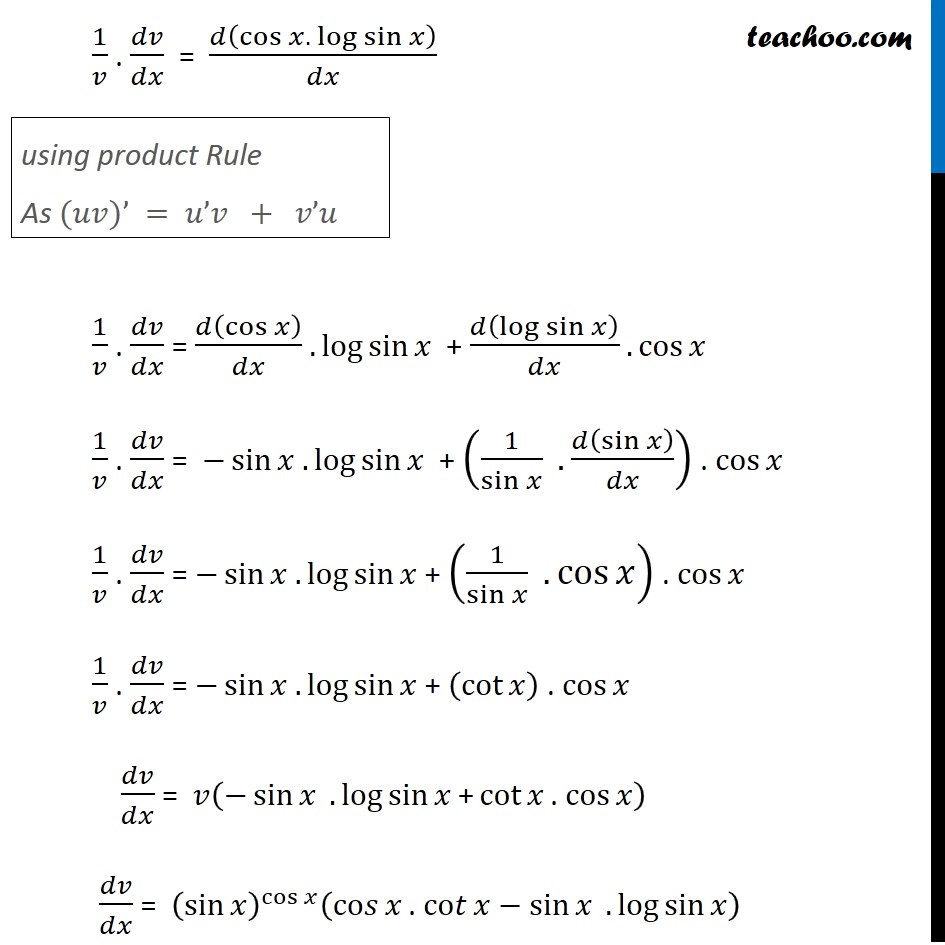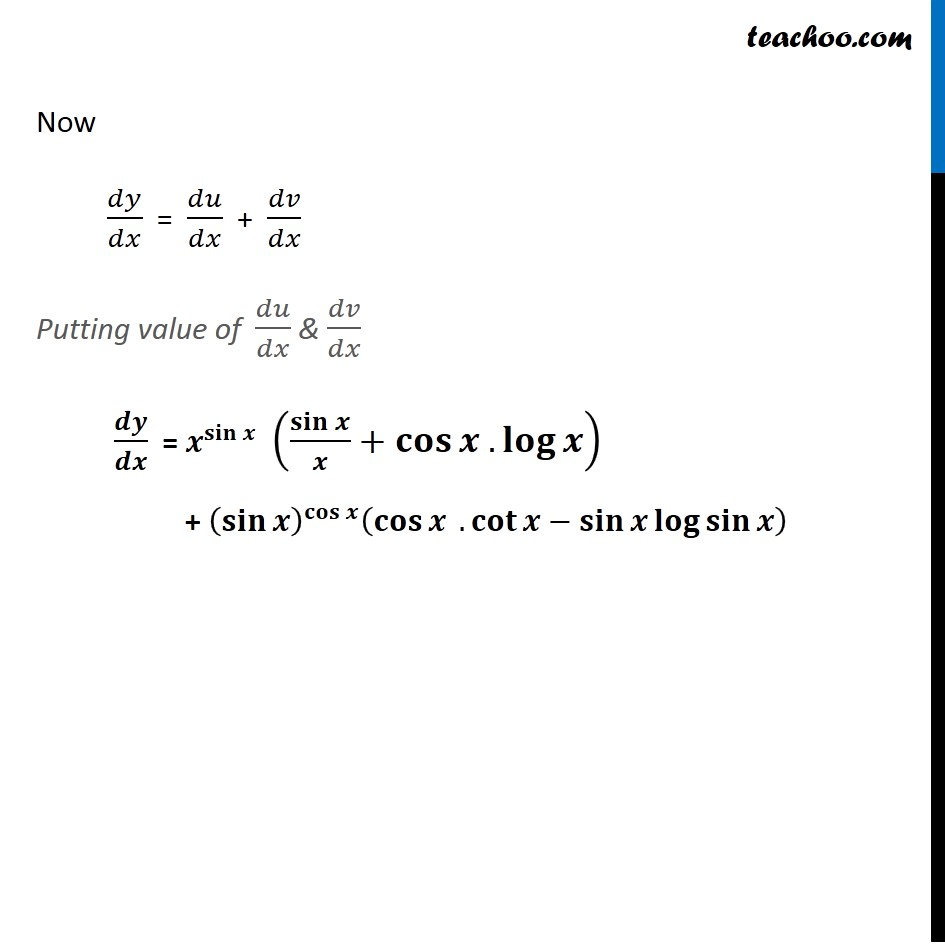1. Chapter 5 Class 12 Continuity and Differentiability (Term 1)
2. Serial order wise
3. Ex 5.5

Transcript

Ex 5.5, 9 Differentiate the functions in, 𝑥^sin⁡𝑥 + 〖(sin⁡𝑥)〗^cos⁡𝑥 Let y = 𝑥^sin⁡𝑥 + 〖(sin⁡𝑥)〗^cos⁡〖𝑥 〗 Let 𝑢 =𝑥^sin⁡𝑥 & 𝑣 =〖(sin⁡𝑥)〗^cos⁡𝑥 ∴ 𝑦 = 𝑢 + 𝑣 Differentiating both sides 𝑤.𝑟.𝑡.𝑥. 𝑑𝑦/𝑑𝑥 = (𝑑 (𝑢 + 𝑣))/𝑑𝑥 𝑑𝑦/𝑑𝑥 = 𝑑𝑢/𝑑𝑥 + 𝑑𝑣/𝑑𝑥 Calculating 𝒅𝒖/𝒅𝒙 𝑢 =𝑥^sin⁡𝑥 Taking log both sides log⁡𝑢=log⁡〖𝑥^sin⁡𝑥 〗 log⁡𝑢= sin⁡𝑥. log⁡〖 𝑥〗 Differentiating both sides 𝑤.𝑟.𝑡.𝑥. (𝑑(log⁡𝑢))/𝑑𝑥 = 𝑑(sin⁡𝑥. log⁡〖 𝑥〗 )/𝑑𝑥 (𝑑(log⁡𝑢))/𝑑𝑥 . 𝑑𝑢/𝑑𝑢 = 𝑑(sin⁡𝑥. log⁡〖 𝑥〗 )/𝑑𝑥 1/𝑢 . 𝑑𝑢/𝑑𝑥 = 𝑑(sin⁡𝑥 . log⁡〖 𝑥〗 )/𝑑𝑥 (As 𝑙𝑜𝑔⁡(𝑎^𝑏) = 𝑏 . 𝑙𝑜𝑔⁡𝑎) Using product Rule As (𝑢𝑣)’ = 𝑢’𝑣 + 𝑣’𝑢 1/𝑢 . 𝑑𝑢/𝑑𝑥 = 𝑑(sin⁡𝑥 )/𝑑𝑥 . log⁡𝑥 + 𝑑(log⁡𝑥 )/𝑑𝑥 . sin 𝑥 1/𝑢 . 𝑑𝑢/𝑑𝑥 = cos⁡𝑥 . log⁡𝑥 + 1/𝑥 . sin 𝑥 1/𝑢 . 𝑑𝑢/𝑑𝑥 = cos⁡𝑥 . log⁡𝑥 + (sin 𝑥" " )/𝑥 𝑑𝑢/𝑑𝑥 = u(cos⁡〖𝑥 .log⁡〖𝑥+ sin⁡𝑥/𝑥〗 〗 ) 𝑑𝑢/𝑑𝑥 = 𝑥^sin⁡𝑥 (cos⁡〖𝑥 .log⁡〖𝑥+ sin⁡𝑥/𝑥〗 〗 ). 𝑑𝑢/𝑑𝑥 = 𝑥^sin⁡𝑥 (〖sin⁡𝑥/𝑥 +cos〗⁡〖𝑥 .log⁡𝑥 〗 ) Calculating 𝒅𝒗/𝒅𝒙 𝑣= (sin⁡𝑥 )^cos⁡𝑥 Taking log both sides log⁡𝑣 = log⁡〖 〖. sin〗⁡𝑥〗^(cos 𝑥) log⁡𝑣=cos⁡𝑥. log sin⁡𝑥 Differentiating both sides 𝑤.𝑟.𝑡.𝑥. (𝑑(log⁡𝑣))/𝑑𝑥 = 𝑑(cos⁡𝑥. log sin⁡𝑥 )/𝑑𝑥 (𝑑(log⁡𝑣))/𝑑𝑥 . 𝑑𝑣/𝑑𝑣 = 𝑑(cos⁡𝑥. log sin⁡𝑥 )/𝑑𝑥 (𝑑(log⁡𝑣))/𝑑𝑣 . 𝑑𝑣/𝑑𝑥 = 𝑑(cos⁡𝑥. log sin⁡𝑥 )/𝑑𝑥 (As 𝑙𝑜𝑔⁡(𝑎^𝑏) = 𝑏 . 𝑙𝑜𝑔⁡𝑎) 1/𝑣 . 𝑑𝑣/𝑑𝑥 = 𝑑(cos⁡𝑥. log sin⁡𝑥 )/𝑑𝑥 1/𝑣 . 𝑑𝑣/𝑑𝑥 = 𝑑(cos⁡𝑥 )/𝑑𝑥 . log⁡sin⁡𝑥 + 𝑑(log⁡sin⁡𝑥 )/𝑑𝑥 . cos⁡𝑥 1/𝑣 . 𝑑𝑣/𝑑𝑥 = −sin⁡𝑥 . log⁡sin⁡𝑥 + (1/sin⁡𝑥 . 𝑑(sin⁡𝑥 )/𝑑𝑥) . cos⁡𝑥 1/𝑣 . 𝑑𝑣/𝑑𝑥 = −sin⁡𝑥 . log⁡sin⁡𝑥 + (1/sin⁡𝑥 .cos⁡𝑥 ) . cos⁡𝑥 1/𝑣 . 𝑑𝑣/𝑑𝑥 = −sin⁡𝑥 . log⁡sin⁡𝑥 + (cot⁡𝑥 ) . cos⁡𝑥 𝑑𝑣/𝑑𝑥 = 𝑣(−sin⁡𝑥 " " . log⁡sin⁡𝑥" +" cot⁡𝑥 ". " cos⁡𝑥) 𝑑𝑣/𝑑𝑥 = (sin⁡𝑥 )^cos⁡𝑥 (co𝑠⁡𝑥 ". " co𝑡⁡𝑥−sin⁡𝑥 " " . log⁡sin⁡𝑥) using product Rule As (𝑢𝑣)’ = 𝑢’𝑣 + 𝑣’𝑢 Now 𝑑𝑦/𝑑𝑥 = 𝑑𝑢/𝑑𝑥 + 𝑑𝑣/𝑑𝑥 Putting value of 𝑑𝑢/𝑑𝑥 & 𝑑𝑣/𝑑𝑥 𝒅𝒚/𝒅𝒙 = 𝒙^𝐬𝐢𝐧⁡𝒙 (𝐬𝐢𝐧⁡𝒙/𝒙+𝐜𝐨𝐬⁡〖𝒙 .𝐥𝐨𝐠⁡𝒙 〗 ) + (𝐬𝐢𝐧⁡𝒙 )^𝐜𝐨𝐬⁡𝒙 (𝐜𝐨𝐬⁡𝒙 .𝐜𝐨𝐭⁡𝒙−𝐬𝐢𝐧⁡𝒙 𝐥𝐨𝐠⁡𝐬𝐢𝐧⁡𝒙 )